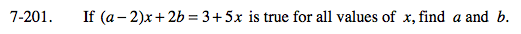### Home > CALC > Chapter 7 > Lesson 7.4.3 > Problem7-201

7-201.

If (a − 2)x + 2b = 3 + 5x is true for all values of x, find a and b. Homework Help ✎Notice that both sides of the equal sign are in mx + b form. Equate the m's and equate the b's.

(a − 2) = 5
(2b) = 3
Find a and b.